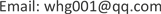1. 引言

ROF模型中的能量泛函分为逼近项和正则项两部分，其中，逼近项用于去除噪声，保证去噪后的图像与原图像的相似性，而正则项则防止去噪过强，起到抑制噪声的作用，因此，需要选取适当的权重参数用于平衡这两项的关系，目前，国内外许多学者都已经提出了一些权重确定方法，具体有无偏预测风险估计器  (URPE)，局部方差估计  ，基于Morozov偏差原理  选取参数等方法。本文基于全变差正则化模型，利用Chambolle对偶投影算法   ，提出了一种参数可以随着迭代算法自适应地改变的新方法，可根据图像的先验信息，能够自适应的选取合适的参数，进而保证去噪后的图像能够保留更多的细节信息。

2. 模型的建立2.1. ROF模型

ROF模型的基本思想是将图像处理问题看成一个能量系统，其中，不含噪声的图像能量相对比较小。当图像被噪声污染后，图像就变得不光滑，其能量也就相应的增大了很多，通过这种思想，就将图像去噪问题转化成了一个能量最小化问题，之后，再通过变分法进行求解。模型如下

min u ∈ B V ( Ω ) E ( u ) = J ( u ) + 1 2 λ ‖ u − f ‖ 2 (1)

2.2. Chambolle去噪对偶模型

0 ∈ ∂ J ( u ) + λ ( u − f ) (2)

u ∈ ∂ J * ( ( u − f ) / λ ) (3)

J ∗ = χ K ( v ) = { 0 v ∈ K + ∞ 其 他 (4)

f λ ∈ f − u λ + 1 λ ∂ J * ( f − u λ )

3. 模型的求解3.1. 投影算法

min ω E ( ω ) = 1 2 ‖ ω − λ f ‖ 2 2 + λ J * ( ω ) (5)

J * 由(5)式已给出，我们可以推导出 ω 一定是 λ f 在凸集K上的正交投影，若 P K λ 表示在集合K上的

u = f − P K λ ( f ) (6)

min p { ‖ λ d i v p − f ‖ 2 : p ∈ Y , | P i , j | 2 − 1 ≤ 0 , ∀ i , j = 1 , ⋯ , N } (7)

− ( ∇ ( λ d i v p − f ) ) i , j + α i , j p i , j = 0 (8)

p i , j n + 1 = p i , j n + τ ( ( ∇ ( d i v p n − f λ ) ) i , j − | ( ∇ ( d i v p n − f λ ) ) i , j | ⋅ p i , j n ) (9)

3.2. BP神经网络

BP神经网络在机构上类似于多层感知器，是一种多层前馈神经网络，在结构上具有三层或三层以上神经元，包括输入层、中间层、和输出层。上下层之间实现全连接，而每层神经元之间无链接。当一对学习样本提供给网络后，神经元的激活值从输入层经各中间层向输出层传播，在输出层的各神经元获得网络的输入相应。

BP网络学习规则

1) 初始化。给每个连接权值 w i , j , v j t 、阈值 θ j 和 γ t 赋予区间 ( − 1 , 1 ) 内是随机值。

2) 随机选取一组输入和目标样本 P k = ( a 1 k , a 2 k , ⋯ , a n k ) , T k = ( S 1 k , S 2 k , ⋯ , S n k ) 提供给网络。

3) 用输入样本 P k = ( a 1 k , a 2 k , ⋯ , a n k ) 连接权 w i , j 和阈值 θ j 计算中间层各单元的输入 s j ，然后用 s j 通过传递函数计算中间各层单元的输出 b j 。

s j = ∑ i = 1 n w i j a i − θ j ,     b j = f ( s j ) ,     j = 1 , 2 , ⋯ , p

4) 利用中间层的输出 b j 、连接权 v j t 和阈值 γ t 计算输出层各单元的输出 L t ，然后通过传递函数计算输出层各单元的响应 C t 。

5) 利用网络目标向量 T k = ( y 1 , y 2 ⋯ , y q ) ，网络的实际输出 C t ，计算输出层的各单元一般化误差 d t k 。再利用一般化误差和各单元的输出来修正连接权和阈值。直到m个训练样本训练完毕。

4. 数值实验与分析

Image quality index compariso

BABOO0.523130.02791540.534130.09057793
BOAT0.471729.018475080.489528.99801813
BIRD0.395227.686664360.408427.78572117

5. 结论